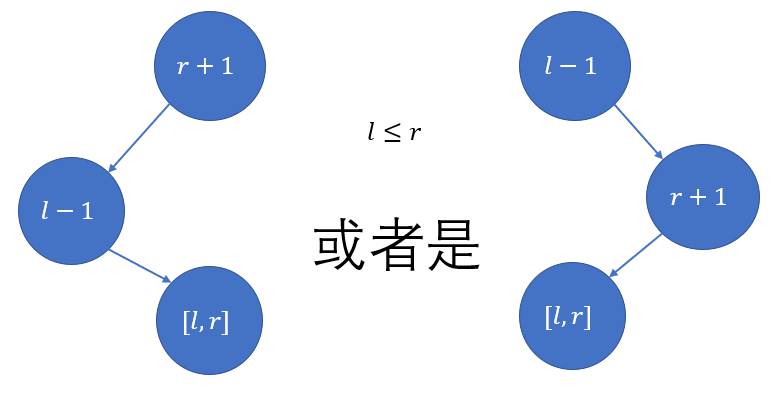# splay学习笔记3 文艺平衡树（伪解题报告）【splay】【平衡树】

首先，区间翻转用splay来做真的是不二选择，感觉现在已经把splay磨得差不多了。这个题其实就是像线段树一样打上lazy-tag来控制时间复杂度的思想，每次访问到一个节点就一定要pushdown一下。

这个题最难想的是如何控制区间翻转，我们联想一个完全二叉树和归并排序，如果要让一个序列倒转过来，那么把它分成$log_2n$段，分别翻转，是没有问题的。但是设这个区间长度为$l$，那么每做一次的复杂度就是$lm$，体现不出优化，那么我们用lazy-tag，只有在访问时才进行翻转操作。

根据完全二叉树的性质，我们如果要把一个区间翻转，就要把它构造成一棵二叉树，将它逐层翻转（左右子树交换）。依照splay和BST的性质，我们可以将这个区间卡到l-1和r+1之间，像下图这样：这样我们就构造出了一棵$[l,r]$的子树，我们对新开发出来的$[l,r]$子树进行翻转就可以了。

Tip：如果要求翻转$[1,n]$怎么办呢？我们就需要分别构造一个0和一个n+1来缓冲这一过程。

不过还有一个比较严重的问题：树翻转以后，不满足BST的性质了，也就是左孩子小于根小于右孩子。所以此时的编号就只是编号，用作最终的中序遍历输出结果的。那么我们怎么找$[l,r]$的区间呢？

由普通平衡树这一题我们了解到，splay是方便查询排名的，我们找目前的排名，找的不是编号，而是位置——区间第几个。我们由BST的性质知道排名小的在左边，我们如果要调用区间第$k$个数，就要在BST中找排名为$k$的数（实际上这一题因为有0这个点，是$k+1$）。所以我们所要做的就是把排名为$l-1$和$r+1$的数调整到根节点，直接进行翻转。

最后我们用中序遍历整理所有的lazy-tag，输出当前的顺序（按排名依次输出）。

初始时如果构造一棵树，依次插入不仅要多写函数，而且是一条链，使效率降低不少，我们可以像构造线段树一样递归建树（见代码注释）

## Code：

#include<cstdio>
#include<cstring>
struct node
{
int key;//存编号
int weight;//存大小
node *s;
bool r;
node(int key)
{
r=false;
this->key=key;
weight=1;
s=NULL;
s=NULL;
}
node()
{
r=false;
s=NULL;
s=NULL;
}
int getw()//访问这棵树的大小
{
if(!this)
return 0;
return weight;
}
void maintain()//维护大小
{
weight=1+s->getw()+s->getw();
}
int getd(int x)//判断应该往左还是往右或者已经找到
{//往左返回0，往右返回1
if(s->getw()+1==x)
return -1;
return s->getw()<x;
}
};
node *root=NULL;
void pushdown(node *&rt)
{
if(!rt)
return;
if(rt->r)
{
node *tmp=rt->s;
rt->s=rt->s;
rt->s=tmp;
if(rt->s)
rt->s->r^=rt->r;
if(rt->s)
rt->s->r^=rt->r;
}
rt->r=false;//记得清零QAQ
return;
}
void Rotate(node *&rt,int dir)//旋转
{
node *tmp=rt->s[dir];
rt->s[dir]=tmp->s[dir^1];
tmp->s[dir^1]=rt;
rt->maintain();
tmp->maintain();
rt=tmp;
return;
}

void splay(node *&rt,int x)
{
pushdown(rt);
int d1=rt->getd(x);
if(d1==-1)
return;
x-=d1*(1+rt->s->getw());//如果是右子树就减去左子树的大小
pushdown(rt->s[d1]);
if(rt->s[d1]->getd(x)==-1)
{
Rotate(rt,d1);
pushdown(rt);
return;
}

int d2=rt->s[d1]->getd(x);

x-=d2*(1+rt->s[d1]->s->getw());//同上
splay(rt->s[d1]->s[d2],x);
if(d1==d2)//zig-zig或zag-zag
{
Rotate(rt,d1);
pushdown(rt);
Rotate(rt,d1);
pushdown(rt);
return;
}
else//zig-zag或zag-zig
{
Rotate(rt->s[d1],d2);
pushdown(rt->s[d1]);
Rotate(rt,d1);
pushdown(rt);
return;
}
}

void turn(int l,int r)
{
//先将r+1提到根，然后提l-1到根左，这样l-1是r+1的左孩子，l-1的右孩子即为所求
//l-1排第l位，r+1排第r+2位

splay(root,r+2);
splay(root->s,l);
root->s->s->r^=1;
return;
}
int n;
void mid_ord(node *rt)
{
pushdown(rt);
if(rt->s)
mid_ord(rt->s);
if(rt->key&&rt->key<=n)
printf("%d ",rt->key);
if(rt->s)
mid_ord(rt->s);
return;
}
void build(node *&rt,int l,int r)//建立一棵平衡的初始二叉树
{
int mid=l+r>>1;//像线段树一样
rt=new node(mid);
if(l<mid)
build(rt->s,l,mid-1);
if(r>mid)
build(rt->s,mid+1,r);
rt->maintain();
}
int main()
{
int m,a,b;
scanf("%d%d",&n,&m);
build(root,0,n+1);
for(int i=1;i<=m;i++)
{
scanf("%d%d",&a,&b);
turn(a,b);
}
mid_ord(root);
printf("\n");
return 0;
}


### 说点什么Subscribe

/* */